# 1 Introduction

Part of the work of Martin et al. (2022) required transforming blood pressure measurement into percentiles based on published norms. This work was complicated by the fact that data for pediatric blood pressure percentiles is sparse and generally only applicable to children at least one year of age and requires height, a commonly unavailable data point in electronic health records for a variety of reasons.

A solution to building pediatric blood pressure percentiles was developed and is presented here for others to use. Inputs for the developed method are:

1. Patient sex (male/female) required
2. Systolic blood pressure (mmHg) required
3. Diastolic blood pressure (mmHg) required
4. Patient height (cm) if known.

Given the inputs, the following logic is used to determine which data sets will be used to inform the blood pressure percentiles. Under one year of age, the data from Gemelli et al. (1990) will be used; a height input is not required for this patient subset. For those at least one year of age with a known height, data from (nhlbi2011exper?) (hereafter referred to as ‘NHLBI/CDC’ as the report incorporates recommendations and inputs from the National Heart, Lung, and Blood Institute [NHLBI] and the Centers for Disease Control and Prevention [CDC]). If height is unknown and age is at least three years, then data from Lo et al. (2013) is used. Lastly, for children between one and three years of age with unknown height, blood pressure percentiles are estimated by the NHLBI/CDC data using as a default the median height for each patient’s sex and age.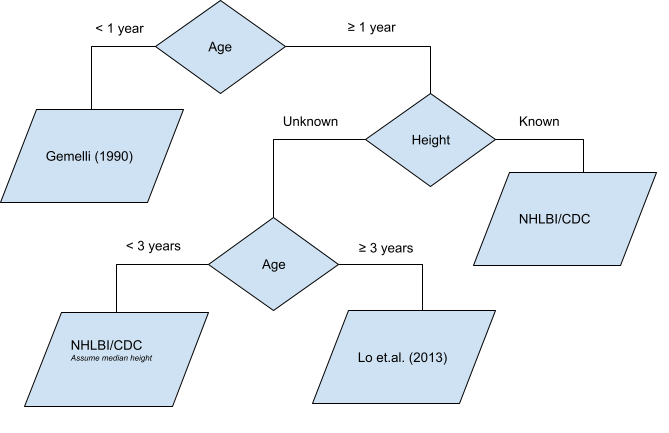# 2 Estimating Pediatric Blood Pressure Distributions

There are two functions provided for working with blood pressure distributions. These methods use Gaussian distributions for both systolic and diastolic blood pressures with means and standard deviations either explicitly provided in an aforementioned source or derived by optimizing the parameters such that the sum of squared errors between the provided quantiles from an aforementioned source and the distribution quantiles is minimized. The provided functions, a distribution function and a quantile function, follow a similar naming convention to the distribution functions found in the stats library in R.

args(p_bp)
## function (q_sbp, q_dbp, age, male, height = NA, height_percentile = 0.5,
##     ...)
## NULL

# Quantile Function
args(q_bp)
## function (p_sbp, p_dbp, age, male, height = NA, height_percentile = 0.5,
##     ...)
## NULL

Both methods expect an age in months and an indicator for sex. If height is missing, e.g., NA, then the default height percentile of 50 will be used as applicable based on the patient’s age group. The end user may modify the default height percentile.

If height is entered, then the height percentile is determined via an LMS method for age and sex using corresponding LMS data from the CDC (more information on LMS methods and data is provided later in this vignette). The parameters for the blood pressure distribution are found in a look up table using the nearest age and height percentile.

## 2.1 Percentiles

What percentile for systolic and diastolic blood pressure is 100/60 for a 44 month old male with unknown height?

p_bp(q_sbp = 100, q_dbp = 60, age = 44, male = 1)
## $sbp_percentile ##  0.7700861 ## ##$dbp_percentile
##  0.72739

Those percentiles would be modified if height was 183 cm:

p_bp(q_sbp = 100, q_dbp = 60, age = 44, male = 1, height = 183)
## $sbp_percentile ##  0.676432 ## ##$dbp_percentile
##  0.8485735

The package can also be used to determine the blood pressure percentiles corresponding to a child of a given height percentile. First find the height quantile using the q_stature_for_age function, and then use this height measurement (provided in centimeters) as the height input for the p_bp function.

ht <- q_stature_for_age(p = 0.90, age = 44, male = 1)
ht
##  105.2123

p_bp(q_sbp = 100, q_dbp = 60, age = 44, male = 1, height = ht)
## $sbp_percentile ##  0.7086071 ## ##$dbp_percentile
##  0.8485735

A plotting method to show where the observed blood pressures are on the distribution function is also provided.

bp_cdf(age = 44, male = 1, height = ht, sbp = 100, dbp = 60)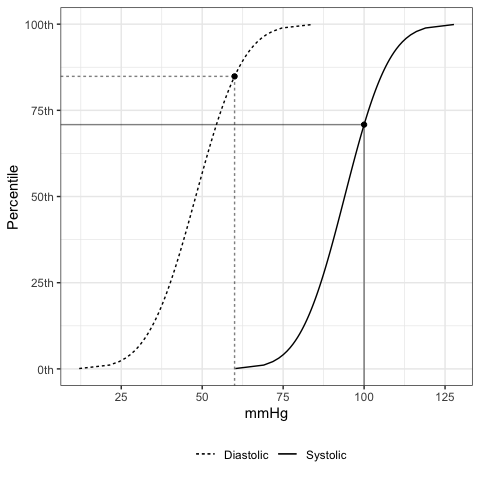Vectors of blood pressures can be used as well. NA values will return NA.

bps <-
p_bp(
q_sbp  = c(100, NA, 90)
, q_dbp  = c(60, 82, 48)
, age    = 44
, male   = 1
, height = ht
)
bps
## $sbp_percentile ##  0.7086071 NA 0.3570545 ## ##$dbp_percentile
##  0.8485735 0.9982486 0.4998928

If you want to know which data source was used in computing each of the percentile estimates you can look at the bp_params attribute:

attr(bps, "bp_params")
##      source male age sbp_mean   sbp_sd dbp_mean   dbp_sd height_percentile
## 147   nhlbi    1  36 94.00085 10.92104 48.00313 11.64367                90
## 1471  nhlbi    1  36 94.00085 10.92104 48.00313 11.64367                90
## 1472  nhlbi    1  36 94.00085 10.92104 48.00313 11.64367                90
str(bps)
## List of 2
##  $sbp_percentile: num [1:3] 0.709 NA 0.357 ##$ dbp_percentile: num [1:3] 0.849 0.998 0.5
##  - attr(*, "bp_params")='data.frame':    3 obs. of  8 variables:
##   ..$source : chr [1:3] "nhlbi" "nhlbi" "nhlbi" ## ..$ male             : int [1:3] 1 1 1
##   ..$age : num [1:3] 36 36 36 ## ..$ sbp_mean         : num [1:3] 94 94 94
##   ..$sbp_sd : num [1:3] 10.9 10.9 10.9 ## ..$ dbp_mean         : num [1:3] 48 48 48
##   ..$dbp_sd : num [1:3] 11.6 11.6 11.6 ## ..$ height_percentile: int [1:3] 90 90 90
##  - attr(*, "class")= chr "pedbp_bp"

## 2.2 Quantiles

If you have a percentile value and want to know the associated systolic and diastolic blood pressures:

q_bp(
p_sbp = c(0.701, NA, 0.36)
, p_dbp = c(0.85, 0.99, 0.50)
, age = 44
, male = 1
, height = ht
)
## $sbp ##  99.75929 NA 90.08611 ## ##$dbp
##  60.07101 75.09035 48.00313

## 2.3 Working With More Than One Patient

The p_bp and q_bp methods are designed accept vectors for each of the arguments. These methods expected each argument to be length 1 or all the same length.

eg_data <- read.csv(system.file("example_data", "for_batch.csv", package = "pedbp"))
eg_data
##           pid age_months male height..cm. sbp..mmHg. dbp..mmHg.
## 1   patient_A         96    1          NA        102         58
## 2   patient_B        144    0         153        113         NA
## 3   patient_C          4    0          62         82         43
## 4 patient_D_1         41    1          NA         96         62
## 5 patient_D_2         41    1         101         96         62

bp_percentiles <-
p_bp(
q_sbp  = eg_data$sbp..mmHg. , q_dbp = eg_data$dbp..mmHg.
, age    = eg_data$age , male = eg_data$male
, height = eg_data$height ) bp_percentiles ##$sbp_percentile
##  0.5533069 0.7680548 0.2622697 0.6195685 0.6101926
##
## $dbp_percentile ##  0.4120704 NA 0.1356661 0.8028518 0.9011263 str(bp_percentiles) ## List of 2 ##$ sbp_percentile: num [1:5] 0.553 0.768 0.262 0.62 0.61
##  $dbp_percentile: num [1:5] 0.412 NA 0.136 0.803 0.901 ## - attr(*, "bp_params")='data.frame': 5 obs. of 8 variables: ## ..$ source           : chr [1:5] "lo2013" "nhlbi" "gemelli1990" "lo2013" ...
##   ..$male : int [1:5] 1 0 0 1 1 ## ..$ age              : num [1:5] 96 144 3 36 36
##   ..$sbp_mean : num [1:5] 100.7 105 89 93.2 93 ## ..$ sbp_sd           : num [1:5] 9.7 10.9 11 9.2 10.7
##   ..$dbp_mean : num [1:5] 59.8 62 54 55.1 47 ## ..$ dbp_sd           : num [1:5] 8.1 10.9 10 8.1 11.6
##   ..$height_percentile: int [1:5] NA 50 NA NA 75 ## - attr(*, "class")= chr "pedbp_bp" Going from percentiles back to quantiles: q_bp( p_sbp = bp_percentiles$sbp_percentile
, p_dbp  = bp_percentiles$dbp_percentile , age = eg_data$age
, male   = eg_data$male , height = eg_data$height
)
## $sbp ##  102 113 82 96 96 ## ##$dbp
##  58 NA 43 62 62

# 3 Blood Pressure Charts

## 3.1 When Height is Unknown or Irrelevant

The following graphic shows the percentile curves by age and sex when height is unknown, or irrelevant (for those under 12 months of age).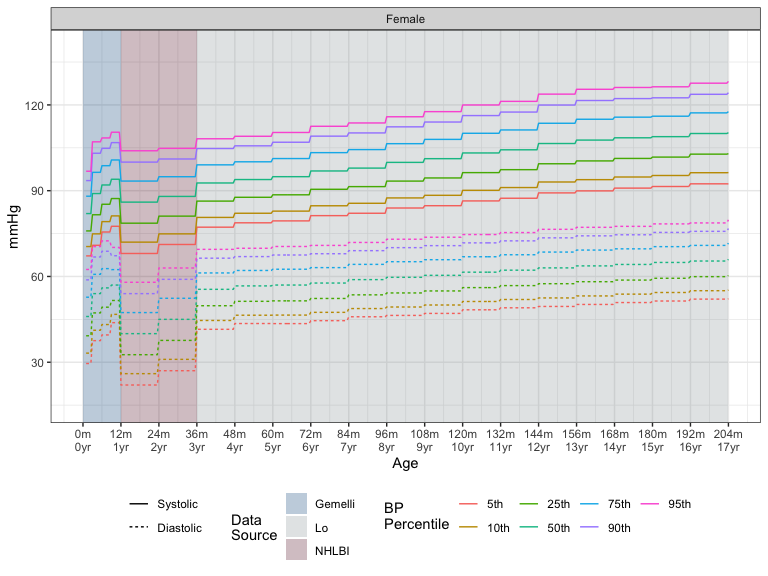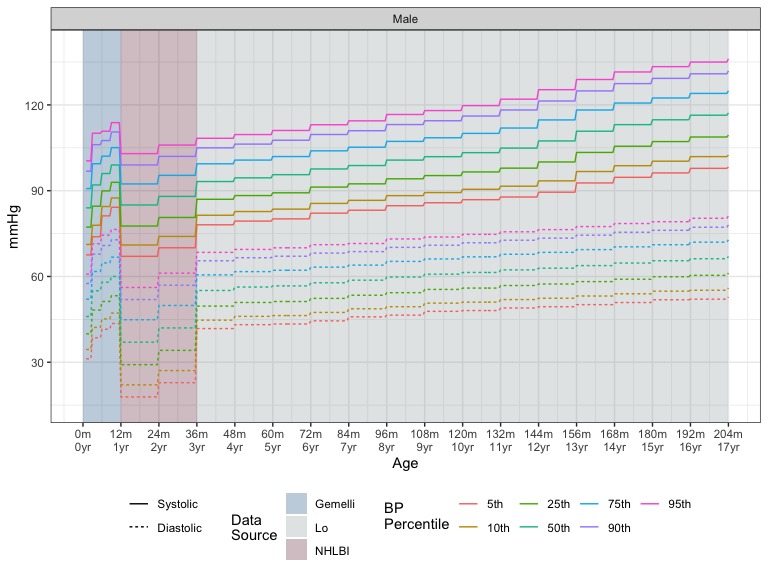## 3.2 Median Blood Pressures – Varying default height percentile

If height is unknown, there will be no difference in the estimated percentile for blood pressures when modifying the default height_percentile with the exception of values for patients between the ages of 12 and 36 months. Patients under 12 months of age have percentiles estimated using data from Gemelli et al. (1990) which does not consider height (length). For patients over 36 months of age data from Lo et al. (2013), which also does not consider height, is used.

The following graphic shows the median blood pressure in mmHg by age when varying the default height percentile used. The colors refer to the height percentile.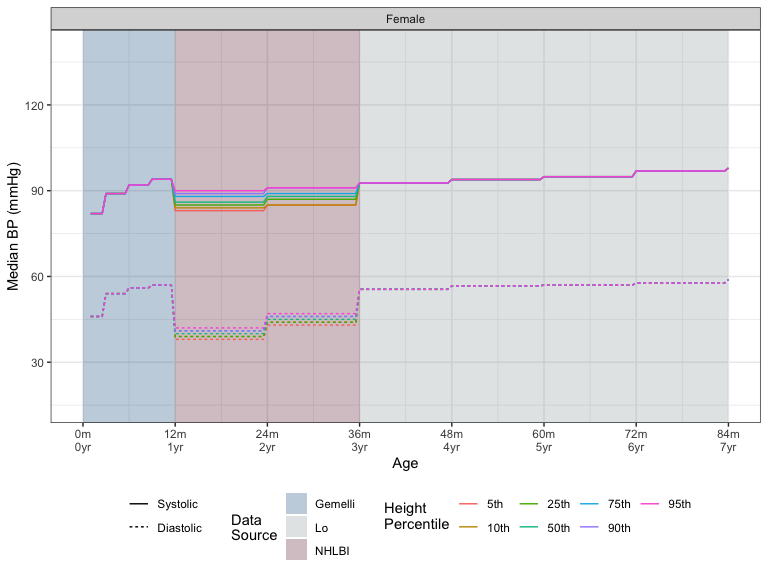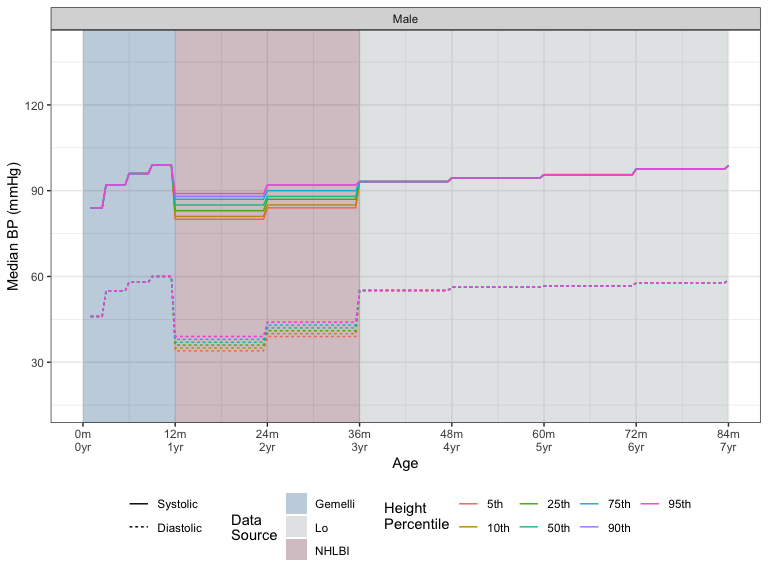## 3.3 Median Blood Pressures for Children with Known Heights

The following chart shows the median blood pressure by age for different heights based on percentiles for age.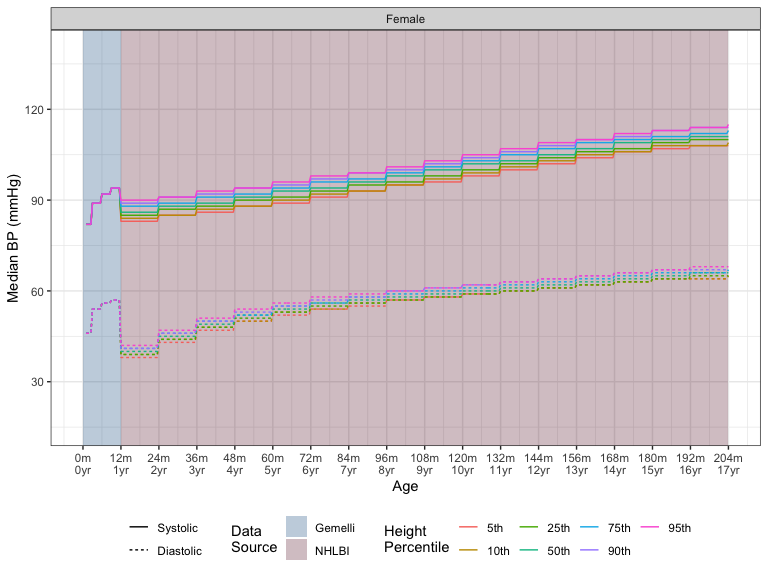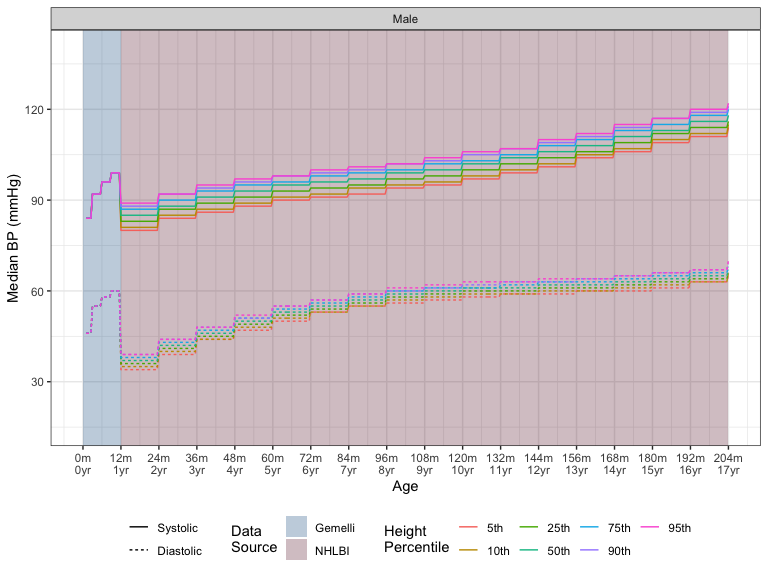# 4 Shiny Application

An interactive Shiny application is also available. After installing the pedbp package and the suggested packages, you can run the app locally via

shiny::runApp(system.file("shinyapps", "pedbp", package = "pedbp"))

The shiny application allows for interactive exploration of blood pressure percentiles for an individual patient and allows for batch processing a set of patients as well.

An example input file for batch processing is provided within the package an can be accessed via:

system.file("example_data", "for_batch.csv", package = "pedbp")

# 5 CDC Growth Charts

Using the Percentile Data Files with LMS values provided by the CDC, we provide eight distribution tools:

1. weight for age for infants
2. length for age for infants
3. weight for length for infants
5. weight for stature
6. weight for age
7. stature for age
8. BMI for age

All lengths/heights are in centimeters, ages in months, and weights in kilograms.

The length-for-age and stature-for-age methods were needed for the blood pressure methods above.

All methods use the published LMS parameters to define z-scores, percentiles, and quantiles for skewed distributions. L is a $$\lambda$$ parameter, the Box-Cox transformation power; $$M$$ the median value, and $$S$$ a generalized coefficient of variation. For a given percentile or z-score, the corresponding physical measurement, $$X,$$ is defined as

$X = \begin{cases} M \left(1 + \lambda S Z \right)^{\frac{1}{\lambda}} & \lambda \neq 0 \\ M \exp\left( S Z \right) & \lambda = 0. \end{cases}$

From this we can get the z-score for a given measurement $$X:$$

$Z = \begin{cases} \frac{\left(\frac{X}{M}\right)^{\lambda} - 1}{\lambda S} & \lambda \neq 0 \\ \frac{\log\left(\frac{X}{M}\right) }{ S } & \lambda = 0. \end{cases}$

Percentiles are determined using the standard normal distribution of z-scores.

For all eight of the noted methods we provide a distribution function, quantile function, and function that returns z-scores.

Estimates for finer differences in age, for example, are possible for these methods than the blood pressure methods. This is due to the permissible linear interpolation of the LMS parameters for the CDC charts whereas the blood pressure assessment is restricted to values within a look up table.

## 5.1 Length and Stature For Age

A 13 year old male standing 154 cm tall is in the 39.51th percentile:

p_stature_for_age(q = 154, age = 13 * 12, male = 1L)
##  0.3951253

To find the height corresponding to the 50th, 60th, and 75th percentiles for height for 9.5-year old girls:

q_stature_for_age(p = c(0.50, 0.60, 0.75), age = 9.5 * 12, male = 0L)
##  135.4252 137.0665 139.8316

If you want the standard score for a percentile, you can use qnorm around p_stature_for_age, or simply call z_stature_for_age.

qnorm(p_stature_for_age(q = 154, age = 13 * 12, male = 1L))
##  -0.2659852
z_stature_for_age(q = 154, age = 13 * 12, male = 1L)
##  -0.2659852

A length/height for age chart based on the CDC data: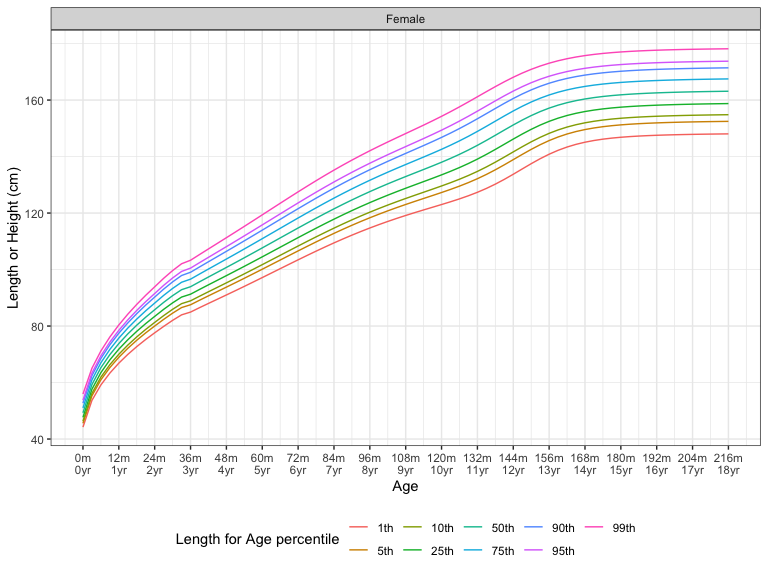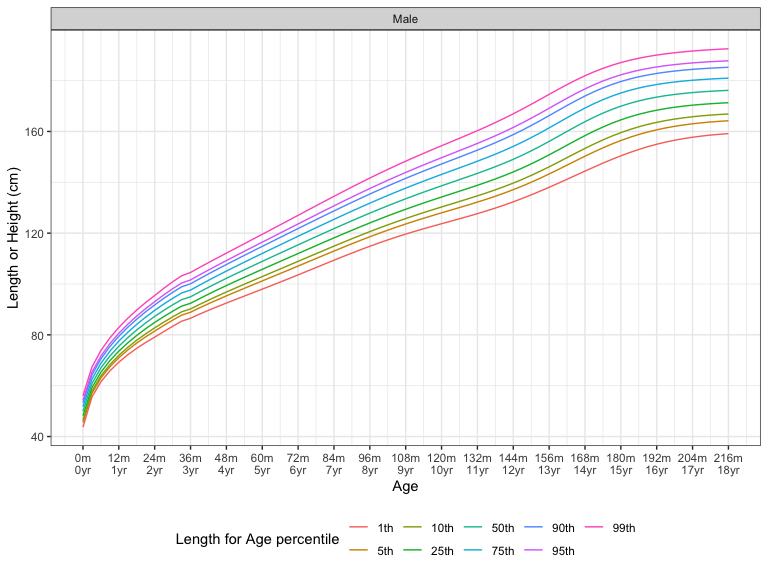## 5.2 Weight for Age

There are two methods for determining weight for age both based on CDC National Center for Health Statistics data: one for infants (weighed laying flat) up to 36 months (“Growth Charts - Data Table of Infant Weight-for-Age Charts” 2001), and one for children (weighed on a standing scale) over 24 months (“Growth Charts - Data Table of Weight-for-Age Charts” 2001).

A 33 pound ( 14.97 kg) 4 year old male is in the 24.11th percentile.

p_weight_for_age(33 * 0.453592, age = 4 * 12, male = 1)
##  0.2410771

The 20th percentile weight for an 18 month old infant female is 10.07575 kg.

round(q_weight_for_age_inf(p = 0.2, age = 18, male = 0), 5)
##  10.07575

## 5.3 Weight for Length or Stature

Similar to weight-for-age, there are two methods for determining weight-for-length. Both methods utilize data from the CDC National Center for Health Statistics. The first method is used for infants under 36 months (used for children who are measured laying flat), and the second is used for child over 24 months (used for children able to be measured while standing up). The overlapping range between the methods will differ.

The median weight for a 95 cm long infant 14.2224238 kg, whereas the median weight for a 95 cm tall child is 14.4183484 kg.

q_weight_for_length_inf(0.5, 95, 1)
##  14.22242
q_weight_for_stature(0.5, 95, 1)
##  14.41835

A 5.8 kg, 61 cm long female infant is in the 0.2977671 weight percentile.

p_weight_for_length_inf(5.8, 61, 0)
##  0.2977671

## 5.4 BMI for Age

For a twelve year old, a BMI of 22.2 corresponds to the 87.28th BMI percentile for a female, and the 90.36th BMI percentile for a male.

p_bmi_for_age(q = 22.2, age = c(144, 144), male = c(0, 1))
##  0.8727830 0.9036248

The median BMI values for a 10 year old male and females are:

q_bmi_for_age(p = 0.5, age = c(120, 120), male = c(1, 0))
##  16.62461 16.83800

A 10 month old male has a median head circumference of 45.6670663 cm.

A head circumference of 42 cm for an 8 month old female is in the 11.29397th percentile.

q_head_circ_for_age(0.5, 10, 1)
##  45.66707
##  0.1129397

## 6.1 Estimating Gaussian Mean and Standard Deviation

The NHLBI data for blood pressures provided values in percentiles. To get a mean and standard deviation that would work well for estimating other percentiles and quantiles via a Gaussian distribution we optimized for values of the mean and standard deviation such that for the provided quantiles $$q_i$$ at the $$p_i$$ percentiles and $$X \sim N\left(\mu, \sigma\right)$$,

$\sum_{i} \left(\Pr(X \leq q_i) - p_i \right)^2,$

was minimized. The NHLBI data is provided to the end user.

data(list = "nhlbi_bp_norms", package = "pedbp")
str(nhlbi_bp_norms)
## 'data.frame':    952 obs. of  6 variables:
##  $male : int 0 0 0 0 0 0 0 0 0 0 ... ##$ age              : num  12 12 12 12 12 12 12 12 12 12 ...
##  $height_percentile: int 5 5 5 5 10 10 10 10 25 25 ... ##$ bp_percentile    : int  50 90 95 99 50 90 95 99 50 90 ...
##  $sbp : int 83 97 100 108 84 97 101 108 85 98 ... ##$ dbp              : int  38 52 56 64 39 53 57 64 39 53 ...

For an example of how we fitted the parameters:

d <- nhlbi_bp_norms[nhlbi_bp_norms$age == 144 & nhlbi_bp_norms$height_percentile == 50, ]
d <- d[d$male == 0, ] d ## male age height_percentile bp_percentile sbp dbp ## 321 0 144 50 50 105 62 ## 322 0 144 50 90 119 76 ## 323 0 144 50 95 123 80 ## 324 0 144 50 99 130 88 est_norm(q = d$sbp, p = d$bp_percentile / 100) ## mean sd ## 105.00091 10.92092 est_norm(q = d$dbp, p = d$bp_percentile / 100) ## mean sd ## 61.99821 10.94227 bp_parameters[bp_parameters$male == 0 & bp_parameters$age == 144 & bp_parameters$height_percentile == 50, ]
##    source male age sbp_mean   sbp_sd dbp_mean   dbp_sd height_percentile
## 89  nhlbi    0 144 105.0009 10.92092 61.99821 10.94227                50
## NA   <NA>   NA  NA       NA       NA       NA       NA                NA

The est_norm method comes with a plotting method too. The provided quantiles are plotted as open dots and the fitted distribution function is plotted to show the fit.

plot( est_norm(q = d$dbp, p = d$bp_percentile / 100) )